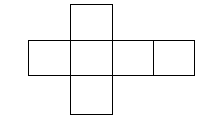# CBSE Class 7 Mathematics Recognition of Solids MCQs

## MCQ for Class 7 Mathematics Recognition of Solids

Class 7 Mathematics students should refer to the following multiple-choice questions with answers for Recognition of Solids in standard 7. These MCQ questions with answers for Grade 7 Mathematics will come in exams and help you to score good marks

### Recognition of Solids MCQ Questions Class 7 Mathematics with Answers

Question: Which of the solids has the maximum number of vertices

a) cuboid

b) pyramid

c) cylinder

d) cone

Question: The curved face of a cylinder can be opened up as a

a) rectangle

b) none of these

c) triangle

d) circle

Question: The angle between two adjacent edges of a cube and cuboid is

a) 900

b) 450

c) 1200

d) 600

Question: A cube is a prism with ________ square faces

a) 6

b) 8

c) 4

Question: A cuboid is a prism with _______ rectangular faces

a) 6

b) 8

c) 4

Question: Which of the following is a polyhedron

a) Prism

b) cylinder

c) cone

Question: The joint between separate faces of a solid is called an

a) edge

b) face

c) vertex

Question: Which of the following has two edges and no comer

a) cylinder

b) Prism

c) cone

Question: A sphere has only 1

a) face

b) vertex

c) edge

Question: A ________ ahs 5 faces, 6 corners and 9 edges

a) triangular prism

b) square pyramid

c) Both

Question: The shape of geometry box

a) cuboid

b) rectangle

c) cube

Question: The shape of a brick

a) cuboid

b) circle

c) Rectangle

d) cube

Question: The shape of a drum

a) cuboid

b) cube

c) cylinder

d) cone

Question: The shape of a playing dice

a) cube

b) sphere

c) cuboid

Question: Which is/are two dimensional figure (i) square (ii) circle (iii) cube (iv) cuboid

a) 1,2,3 only

b) 2,3,4 only

c) All of these

d) 1,2 only

Question: Which one of the following is not a cuboid

a) playing die

b) geometry box

c) briefcase

d) a box of corn flakes

Question: Which one of the following is not a sphere

a) a ring

b) a cricket ball

c) a tennis ball

Question: Which one of the following is not a cylinder

a) a milk carton

b) a mug

c) a beaker

Question: The following figure is the net of aa) cube

b) cuboid

c) Triangular pyramid

d) Pentagonal Prism

## Books recommended by teachers

 CBSE Class 7 Mathematics Integers MCQs
 CBSE Class 7 Mathematics Fractions and Decimals MCQs
 CBSE Class 7 Mathematics Data Handling MCQs
 CBSE Class 7 Mathematics Linear Equations In One Variable MCQs CBSE Class 7 Mathematics Simple Equations MCQs
 CBSE Class 7 Mathematics Lines and angles MCQs CBSE Class 7 Mathematics Parallel Lines and Transversal MCQs
 CBSE Class 7 Mathematics Triangle and its Properties MCQs
 CBSE Class 7 Mathematics Congruence of Triangles MCQs
 CBSE Class 7 Mathematics Rational Numbers MCQs
 CBSE Class 7 Mathematics Perimeter and Area MCQs CBSE Class 7 Mathematics Quadrilaterals and Circles MCQs
 CBSE Class 7 Mathematics Algebraic Expression MCQs
 CBSE Class 7 Mathematics Exponents and Powers MCQs
 CBSE Class 7 Mathematics Linear Symmetry MCQs
 CBSE Class 7 Mathematics Recognition of Solids MCQs CBSE Class 7 Mathematics Symmetry and Visualising Solid Shapes MCQs
 CBSE Class 7 Mathematics All Chapters MCQs